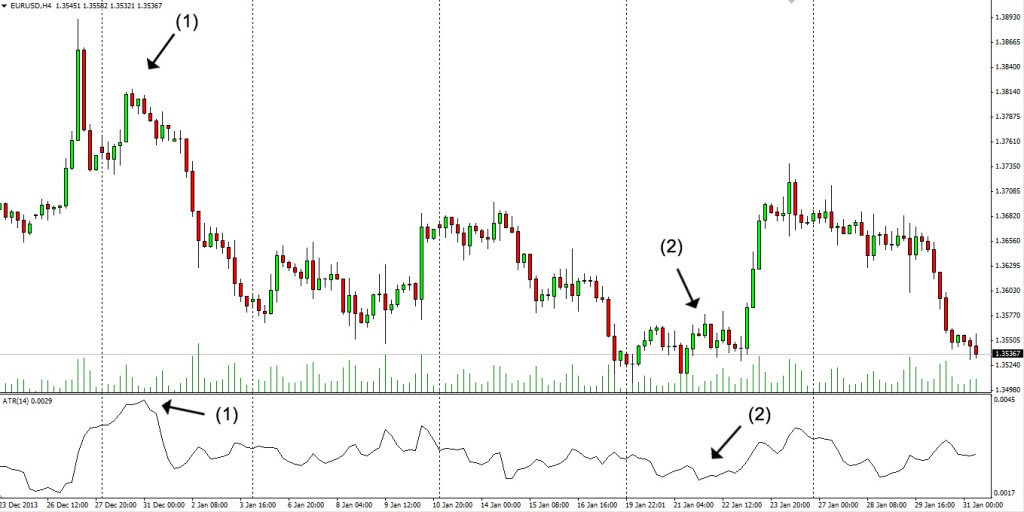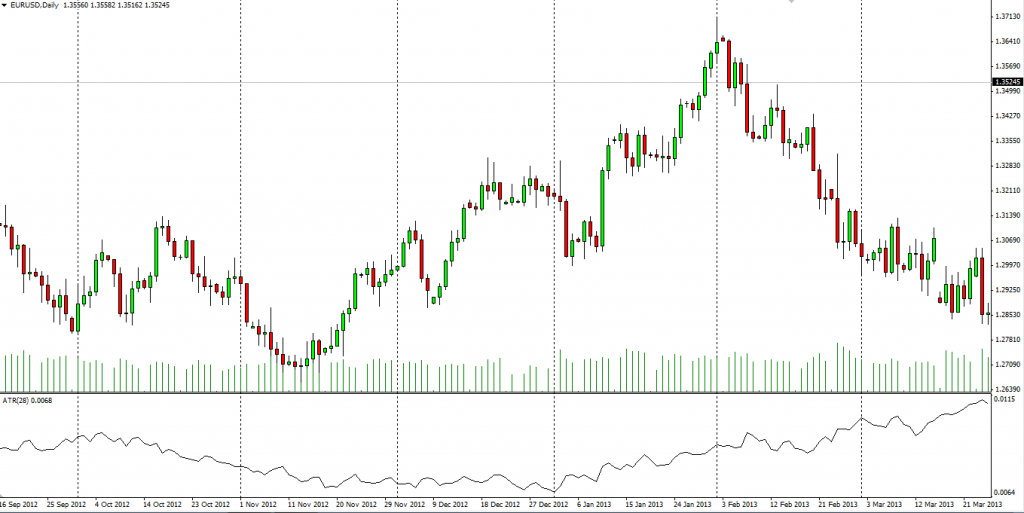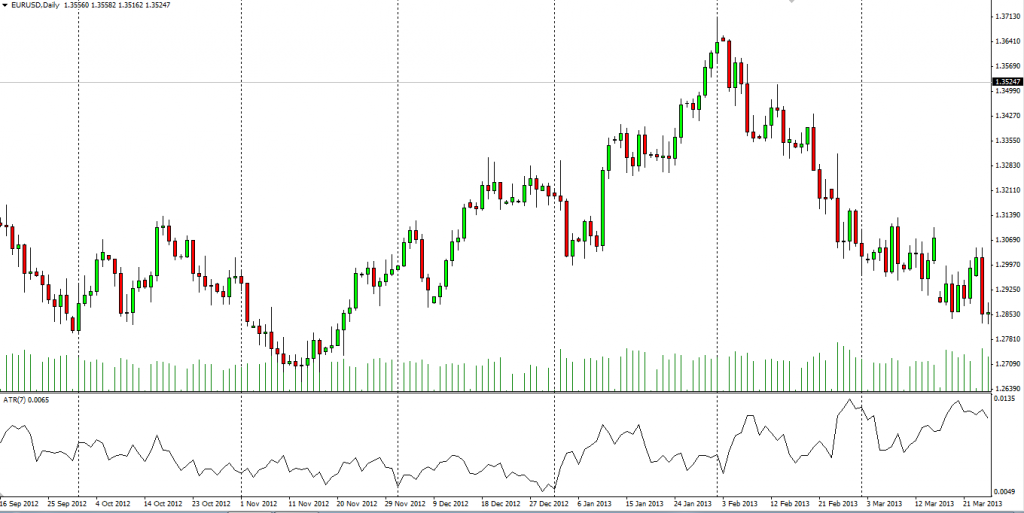# Average True Range

## Average True Range

### This lesson will cover the following

• What is Average True Range
• How is the ATR calculated
• What does it show you

The Average True Range is a volatility indicator measuring how much the price of an asset has moved over a certain number of periods, in other words how volatile the asset is. It was created by J. Welles Wilder and was featured in his book “New Concepts in Technical Trading System”. It was originally designed as a volatility indicator able to capture gaps in commodities, since a volatility formula based solely on the high-low range would miss that movement. However, the ATR can be used for stocks, indexes and currencies as well.

What traders use the ATR for is to determine their profit target and the optimal price level for placing protective stops by predicting how far the asset may move in the future. The Average True Range is most commonly calculated on a 14-period basis, but as with most other indicators, it can be fine-tuned according to each traders unique trading system.

## Best Forex Brokers for United States

The ATR is a directionless indicator, basically a type of moving average of the assets price movement over a certain period of time, which does not indicate the direction of the trend. You can see how the ATR is visualized on a chart on the screenshot below.As you can see, the actual value is plotted in the top left hand corner. Weve used the default 14-period basis, which means that the average price movement over the last 14 four-hour periods is 29 pips. A trader can therefore expect the price to move within the range of 29 pips during the current period, thus giving a hint where his/her profit target and protective stops should be (we will discuss those a bit later in the article).

When the line goes up, in our example at (1), the indicator shows that volatility is rising, while a downward tilted line, just like at (2), indicates falling volatility. Low ATR values, especially for an extended period, are typical when the market trades sideways. Direction of the market trend is irrelevant.

## How is the ATR calculatedThe Average True Range is calculated by estimating the True Range for each of the included periods and then finding their average using a formula, which is shown below.

The True Range is defined as the greatest of the following:

– The difference between the current high and the current low

– The difference between the current high and the previous close in an absolute value

– The difference between the current low and the previous close in an absolute value

The first scenario is used when the current high is above the previous periods high and the current low is below the preceding periods low (the previous candle is engulfed by the current one).

The second and third scenarios are used when a gap has occurred or the current period is engulfed by the previous period. Since Wilder was interested in measuring the distance between two points, and not in the direction of movement, here we use absolute values.

After weve calculated the True Range for each of the periods we have decided to track back, we must now calculate the Average True Range by adding these values and calculating their average (as weve already said, the ATR is a moving average of the TR values).

As mentioned before, the most commonly used and set as default in most trading platforms period setting is 14 periods. After weve estimated the ATR for the initial 14 periods, we must then use the following algorithm to estimate future values:

Current ATR = [(Prior ATR x 13) + Current TR] / 14

## How to trade the ATRYouve already learned that the Average True Range acts as a tool to measure the degree of interest or disinterest in a price movement. This means that inspiring moves are often accompanied by large TRs, especially at the beginning of a move, while weak moves are followed by narrow ranges. This allows us to use this indicator to gauge the enthusiasm behind every move, including breakouts.

For example, a price reversal, accompanied by an increase in the ATR value would suggest strong sentiment toward that move and reinforce the reversal, while weak ATR would suggest to proceed with caution.

This is also true when the price breaks through a support or resistance. If the breakout is supported by a rise in the ATR, it will be most likely a real move, but waning support from the indicator would suggest that the breakout might be false.

### Profit target

Since the ATR measures how much the price of an asset has moved over a certain number of periods, it can be used to predict how much the price will change in the future, giving us a hint where our profit target should stand. If the indicator is showing that the price has moved an average 50 pips during the tracked period and the market has exceeded those 50 pips during the current period, then a price reversal is quite possible.On the screenshot above you can see the price summary of a particular candle and the Average True Range for the same period. With the ATR showing a value of 29 pips, we can assume that at the time of opening of the respective candle we could have expected it to hold in that range, or close to it, as it happened. As we enter a short position, we set our profit target at 29 pips, which we wouldve achieved near the low of the candle.

### Protective stopThe same way the ATR is used for setting a profit target, it can serve as a tool to place your stop loss on the other side of the price action. If the average price range during the last 14 periods is 50 pips, then you can set your protective stop 50 pips below or above the opening of the current period, depending on the type of position you are entering. In the example provided above, we set our stop loss 29 pips above the open price, since this is a short position, as marked by the red line.

Using the Average True Range when placing your stop loss allows you to effectively avoid random market noise. By setting the protective stop far away from the entry point, according to the ATR value, temporary price movements are almost always unable to trigger it and close the position at a loss. Generally, the price move will exceed the ATR value only with a good reason, thus rendering this protective-stop system relatively irrelevant to temporary random price movements.

If however the price action does turn against you, then the ATR value would have provided you with one of the shortest stop loss levels possible that would diminish the negative effect on your account, whilst avoiding random noise.

## Changing settingsAs we know, the most commonly used period used to calculate the ATR is 14 periods, just like with the Relative Strength Index. This setting however is a subject of change according to each traders uniquely tuned trading system. As with each other indicator however, the larger the period of data included, the smoother the line visualizing the indicator will be. Therefore it will produce less false signals, but the ones it does generate, will most likely be lagging the price action too much. On the screenshot below weve illustrated how an ATR calculated on the base of 28 periods (twice more than usual) looks like.Logically, the ATR line will be fluctuating much more each time when reduce the periods of data tracked back. The lower the number of periods, the more sensitive the ATR will be to recent price action and will give faster readings, but at the cost of more false signals. The same price action is pictured below, but with the ATR period tuned down, so that you can compare the two scenarios.As you can see, both of these periods deviating from the default level dont seem so suitable, especially for novice traders. We suggest that you first do extensive testing using the conventional 14-period time frame and then, if you are not satisfied, try out a setting that personally suits you best. But dont forget to scrutinize the new setting as well, before incorporating it in your real-account trading strategy.